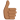# Spaceship Special relativity

## Homework Statement

1. A spaceship travels from Earth to the vicinity of the star that is measured by astronomers on Earth to be six light-years away. The spaceship and its occupants have a total rest mass of 32 000 kg. Assume that the spaceship travels at constant velocity. The time taken as measured by clocks on the spaceship is 2.5 years.
1. (i) Compute the velocity of the spaceship. [3 marks]

2. (ii) How long does the trip take as measured by clocks in the Earth’s

inertial rest frame? [3 marks]

## Homework Equations

x' = γ(x-ut)
t' = γ(t - ux/c2) where the dash frame is the object rest frame, u is the relative speed between frames
γ = 1/√(1-u2/c2)
v' = v-u/1-u2v/c2

## The Attempt at a Solution

So I'm a bit stuck my the first part of the question.
So far I have determined that x = 6ly, t' = 2.5yrs. I'm getting a bit puzzled as to what I'm looking to find. Is it u
or v or are they the same thing in this case? regardless I cannot think of a way in which i have enough variables that allows me to compute a value. Any help would be greatly appreciated!

Doc Al
Mentor
Sometimes you have to chip away at it. See if you can solve for x'. (Hint: You'll need a different version of your first equation.)

I'm getting a bit puzzled as to what I'm looking to find. Is it u
or v or are they the same thing in this case?
You are solving for u. v is irrelevant: there's only one velocity in this problem.

Ok so I'm not sure if this works or not but this is what I've got:
t = γt' and t = x/u.
Combining this gives x/t' = uγ and then expanding this all out and rearranging gives a value for u. Would this method work?

Doc Al
Mentor
Ok so I'm not sure if this works or not but this is what I've got:
t = γt' and t = x/u.
Combining this gives x/t' = uγ and then expanding this all out and rearranging gives a value for u. Would this method work?
That will do it. Good!